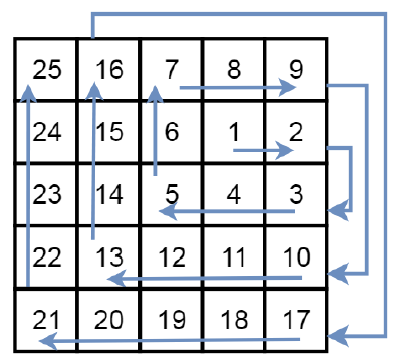# Spiral Matrix III in C++

Suppose we have a 2 dimensional grid with R rows and C columns, we start from (r0, c0) facing east. Here, the north-west corner of the grid is at the first row and column, and the south-east corner of the grid is at the last row and column. We will walk in a clockwise spiral shape to visit every position in this grid. When we are at the outside the boundary of the grid, we continue our walk outside the grid (but may return to the grid boundary later.). We have to find a list of coordinates representing the positions of the grid in the order they were visited. So if the grid is like −Then the arrow will be the path.

To solve this, we will follow these steps −

• Create dirr := [[0,1],[1,0],[0,-1],[-1,0]]

• create a matrix ret, len := 0, dir := 0

• insert (r0, c0) into ret

• while size of ret < R*C

• if dir = 0 or dir = 2, then increase len by 1

• for i in range 0 to len – 1

• r0 := r0 + dirr[dir, 0], c0 := c0 + dirr[dir, 1]

• if r0 in range of 0 to R, and c0 in range 0 to C, then go for next iteration

• insert (r0, c0) into ret

• dir := (dir + 1) mod 4

• return ret

Let us see the following implementation to get better understanding −

## Example

Live Demo

#include <bits/stdc++.h>
using namespace std;
void print_vector(vector<vector<auto> > v){
cout << "[";
for(int i = 0; i<v.size(); i++){
cout << "[";
for(int j = 0; j <v[i].size(); j++){
cout << v[i][j] << ", ";
}
cout << "],";
}
cout << "]"<<endl;
}
int dirr = {{0, 1}, {1, 0}, {0, -1}, {-1, 0}};
class Solution {
public:
vector<vector<int>> spiralMatrixIII(int R, int C, int r0, int c0) {
vector < vector <int> > ret;
int len = 0;
int dir = 0;
ret.push_back({r0, c0});
while(ret.size() < R * C){
if(dir == 0 || dir == 2) len++;
for(int i = 0; i < len; i++){
r0 = r0 + dirr[dir];
c0 = c0 + dirr[dir];
if(r0 < 0 || c0 < 0 || c0 >= C || r0 >= R) continue;
ret.push_back({r0, c0});
}
dir = (dir + 1) % 4;
}
return ret;
}
};
main(){
Solution ob;
print_vector(ob.spiralMatrixIII(5,5,1,3));
}

### Input

5

5

1

3

## Output

[[1,3],[1,4],[2,4],[2,3],[2,2],[1,2],[0,2],[0,3],[0,4],[3,4],[3,3],[3,2],[3,1],[2,1],[1,1],[0,1],[4,4],[4,3],[4,2],[4,1],[4,0],[3,0],[2,0],[1,0],[0,0]]

Updated on: 30-Apr-2020

238 Views